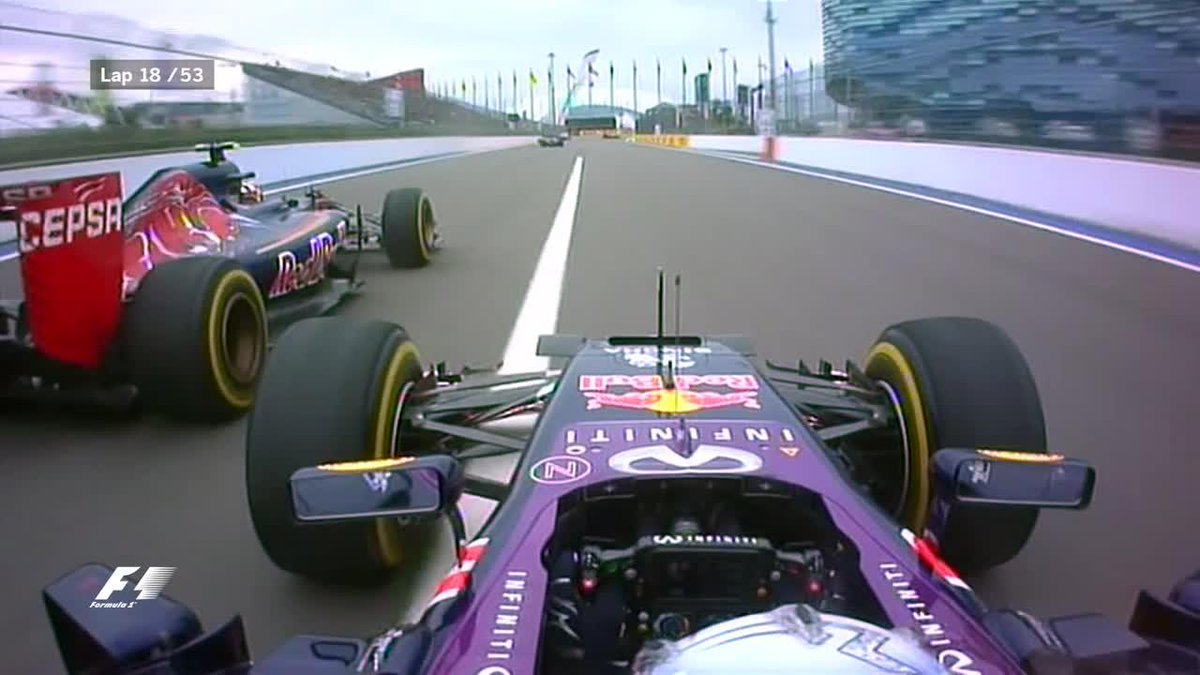In [ ]:
import R_audience as data_enthusiasts# “My model shows…”¶

## communicating data science to the management¶

### Ágoston Török, Synetiq¶

@torokagoston @SynetiqLab

# Three stories¶

• Support vector machines
• Ensemble of trees
• Convolutional neural network
In [ ]:
%pylab inline
import pandas as pd

data1 = np.loadtxt('/storage/wheel_trajectory_data.csv')
data2 = pd.read_csv('/storage/eeg_data.csv')
data3 = pd.read_csv('/storage/driven_data_challenge.csv')# The problem¶

• The car in front of you can make unexpected maneuvers
• We got information too late (steering backlash, vehicle inertia, tire stiffness)
• Vehicle2vehicle communication system
• Quick classification to danger and no-danger

*Ágoston Török, Krisztián Varga, Jean-Marie Pergandi, Pierre Mallet, Ferenc Honbolygó, Valéria Csépe, Daniel Mestre

# Solution¶

• One Class SVM
• Finds the most optimal hyperplane that differentiates in-lier and outlier cases
• Prediction based on Support Vectors (sparsity)

$k(x,G)=\exp\left(-\frac{\|x-G\|^{2}}{\sigma^{2}}\right)$

In [ ]:
from sklearn.svm import OneClassSVM

# since it is a OC-SVM gridsearch not works. We can
# find a way to minimize the positives, but without seeing
# those positives we cannot be certain that those should be
# excluded.

# this appeared to be the best parameters for the model with < 5% false alarms
model = OneClassSVM(nu=0.05, # sparsity parameter (other models it is C)
gamma=0.25, # kernel width
kernel='rbf', # Gaussian kernel
shrinking=True, # temporarily gets rid of unlikely SV candidates
random_state=21)
model.fit(train_xw)
prediction = model.predict(validation_xw)# Conclusion¶

• Smoother decision function -> 'If it is a no go you will see the signs'
• Sparse representation -> 'We don't have to check each and every cases everytime'# The problem¶

• Biosignals are noisy
• Finding noisy epochs is time consuming
• Sometimes it is rather difficult to define precisely what is noise

*David Tellez, (Agoston Torok)

In :
from keras.models import Model
from keras.layers import Dense, Convolution1D, MaxPooling1D, Input, Flatten
from IPython.display import SVG
from keras.utils.visualize_util import model_to_dot

input_signal = Input(shape=(256, 1))

cl1 = Convolution1D(32,  10, border_mode='same', activation='relu')(input_signal)
cl2 = Convolution1D(32,  6, border_mode='same', activation='relu')(cl1)
mp1 = MaxPooling1D(2, border_mode='same')(cl2)
flat = Flatten()(mp1)
decision = Dense(1, activation='sigmoid')(flat)

model = Model(input=input_signal, output=decision)

SVG(model_to_dot(model, show_shapes=True).create(prog='dot', format='svg'))

Out:

# Conclusion¶

• Neural networks can learn well the non-linear boundaries -> 'I don't have to spend two more months crossvalidating'
• Neural networks require a lot of training data -> 'You do have to gimme more data'
• The architecture is of core importance <-# Problem¶

• You have large amount of noisy data from several sources
• You have human made categorical labels
• Limited time do it

*Agoston Torok, Adam Csapo, Krisztian Varga, Adam Divak

In [ ]:
from sklearn.ensemble import ExtraTreesClassifier

# meta estimator that fits a number of randomized decision trees (a.k.a. extra-trees)
# on various sub-samples of the dataset and use averaging to improve the predictive
# accuracy and control over-fitting.

# XGBoost - features are selected based on previous trees, splits are selected to best differentiate
# Random forest - features are selected at random, splits are selected to best differentiate
# Extremely randomized trees - features and splits are also at random

model = ExtraTreesClassifier(n_estimators=500, # a large amount of estimators
max_depth= 10,  # regularization
n_jobs=-1, # can be parallel
criterion='entropy', # or gini impurity
max_features=80) # so we had a huge number of features# Conclusion¶

• Not only RF or XGBoost - 'You are not a one tool guy/girl'
• Computationally cheap - 'I don't need that X1 AWS after all'
• Feature importance can be defined - 'That number is important'

# Take home messages¶

• Income & understandability
• Being an expert means acknowledging statistical significance
• Businesswise vs datasciencewise solution

# Thank you for your attention!¶

@torokagoston @SynetiqLab

In [ ]: